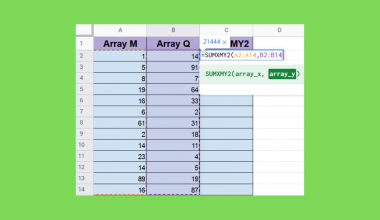How to Fix ‘Circular Dependency Detected’ Error in Google Sheets

The circular dependency detected errors in Google Sheets is due to the formula being within the range you’re referring to or because the input of your formula is dependant on the output.

This is a relatively annoying error to deal with, as Google Sheets returns this error without indicating why it occurred.

There are several reasons why the circular dependency detected errors appears in Google Sheets.

Here are the three main causes:

1. The range selected contains the formula
2. The input of the formula depends on the output
3. Omitting the tab name when selecting data from another tab

These cases may seem complicated, do not worry, as we would use some examples to help you visualize the cause and how to solve it!

The Range Selected Contains the Formula

One of the most common mistakes is when users unknowingly included the formula as part of the range selected.

Let us use a simple example to show you what a circular dependency detected errors in Google Sheets looks like.

Example 1:As seen in this example, the formula in cell A5 is within the selected range. This would cause the formula to return a circular dependency detected error.

To correct this error, we will change the formula’s selected range to only add the numbers in the cells above it, A1:A4.

Example 2:

Let us use an example that is applicable in real life. The circular dependency detected error often appears when attempting to use the FILTER function as well. To understand how the FILTER function works, you can visit our tutorial on it!In this example, there is a circular dependency detected error as the range selected contains the formula itself.

To fix this error, we should exclude the formula from the range making the formula to be =FILTER(A2:B,B2:B>4)

A tip to avoid the circular dependency detected error is to distance the formula away from the range of data to be selected. This would help reduce the mistakes above.

The Input of the Formula Depends on the Output

For those who use more than one formula in Google Sheets, you may come across the circular dependency detected error on multiple formulas altogether.

Example 1:

In this example, we want to find out if these stocks are low or high in quantity and if they need to be restocked or not.

We will be using two IF functions are there are two arguments. Do not hesitate to visit a tutorial on the IF function to better understand how it works!As shown in the image, both of these IF function formulas are having circular dependency detected errors.

The issue is that the formula in cell C3 refers to D3 instead of B3 to check if the quantity left is high or low.

To fix these errors, we would form the IF function argument to check if the stock is high or low to refer to column B instead of column D.As you can see, we have decided that if the quantity of the stock is greater than 20 pieces, it would be “High”.

Hence the correct formula should be:

=IF(B3>20,"High","Low")

Now, the formulas are no longer dependent on each other’s outputs. By correcting only one formula, we can remove the circular dependency detected error from both formulas.

Omitting the Tab Name When Selecting Data from Another Tab

Another common cause for the circular dependence detected error to appear is when you refer to a different tab in your formula and neglect to include the tab name in your reference.

Example 1:

In this example, we will be using the FILTER function to filter and return the rows or columns in a range that meets the specified criteria.

However, the data would be in a different tab.If we do not insert the specific tab name that the data range is in, the circular dependence detected error would appear.This is because the selected range without included the specific tab name would contain the formula itself. This is similar to the first reason stated above.

To fix the error, we would need to specify the particular tab name in the formula.We help direct Google Sheets to the correct tab for the desired data range by specifying the tab name.

You may make a copy of the spreadsheet using the link attached below and try it for yourself:

You now know how to quickly correct this error in your Google Sheets anytime it occurs!

If you are interested to learn how to fix other errors, do not miss out on our tutorial on the five most common formula parse errors in Google Sheets!Our goal this year is to create lots of rich, bite-sized tutorials for Google Sheets users like you. If you liked this one, you'll love what we are working on! Readers receive ✨ early access ✨ to new content.

You May Also LikeHow to Use the COUPNUM Function in Google Sheets

The COUPNUM function in Google Sheets is used to calculate the number of coupons (interest payments) that will…How to Sort Data in Google Sheets

In this article, we’ll learn how to sort data in Google Sheets. We’ll see the built-in functionality in…How to Use ISOWEEKNUM Function in Google Sheets

The ISOWEEKNUM function in Google Sheets is useful for finding out what week a specific date falls into.…How To Rotate Text In Google Sheets

Knowing how to rotate text in Google Sheets is useful if you want to change the rotation of…